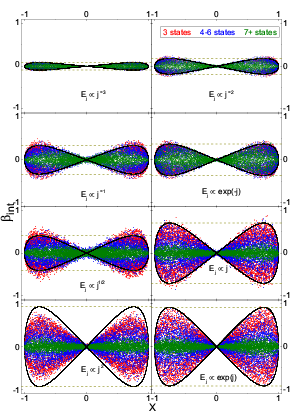# Patterned Energy - Performing Simulations in the Area of Non-Linear Optics

 Shoresh Shafei and Mark Kuzyk in the Physics department at Washington State University are using Origin to perform simulations in the area of non-linear optics. They use Origin's unique project explorer to organize gigabytes of simulation data, Origin's analysis tools for computation, and multi-panel graphing capabilities to present results. The dipole moment "p" of an atom or molecule exposed to intense radiation can be expressed by the following polynomial function of the driving electric field "E" : p = α*E + β*E2 + γ*E3 + ... The non-linear optical coefficients, β and γ, are known as the first and second hyperpolarizabilities (HPs). Shafei and Kuzyk seek to quantify the behavior of these coefficients from the fundamental physics of quantum systems. It is known that, at the off-resonant regime, the HPs are a function of both the transition moments and the energies of quantum states. The energy of states and transition moments for a given system are constrained by what are known as Sum Rules. Previous studies using Monte Carlo simulations to model systems based on the Sum Rules demonstrate that values of the HPs vary continuously between theoretical minima and maxima. Physical measurements, however, have shown that the highest observed HPs are lower, by a factor of 20-30, than the theoretical maxima. In the current study, the classes of energies corresponding to physical models were used in an attempt to provide some explanation for the gap between theoretical and experimental values of the HPs. One of the key findings of the study is shown in the adjacent figure. The figure visualizes the locus of values for the intrinsic first HP, defined as β/βmax, plotted against the normalized |x01| transition moment, X = x01/x01,max. The data are color coded by the number of active states in the system.Locus of values of the first hyperpolarizability (βint) with variations in transition moment (X), subject to energy constraints on the system.

This work was published in the Journal of the Optical Society of America B:
http://www.opticsinfobase.org/josab/abstract.cfm?uri=josab-28-4-882

A pre-publication PDF of the manuscript is archived here:
http://arxiv.org/PS_cache/arxiv/pdf/1101/1101.1041v1.pdf